# 3.2.4.3.1. `sklearn.ensemble`.RandomForestClassifier¶

class `sklearn.ensemble.``RandomForestClassifier`(n_estimators=10, criterion='gini', max_depth=None, min_samples_split=2, min_samples_leaf=1, min_weight_fraction_leaf=0.0, max_features='auto', max_leaf_nodes=None, min_impurity_split=1e-07, bootstrap=True, oob_score=False, n_jobs=1, random_state=None, verbose=0, warm_start=False, class_weight=None)[source]

A random forest classifier.

A random forest is a meta estimator that fits a number of decision tree classifiers on various sub-samples of the dataset and use averaging to improve the predictive accuracy and control over-fitting. The sub-sample size is always the same as the original input sample size but the samples are drawn with replacement if bootstrap=True (default).

Read more in the User Guide.

Parameters: n_estimators : integer, optional (default=10) The number of trees in the forest. criterion : string, optional (default=”gini”) The function to measure the quality of a split. Supported criteria are “gini” for the Gini impurity and “entropy” for the information gain. Note: this parameter is tree-specific. max_features : int, float, string or None, optional (default=”auto”) The number of features to consider when looking for the best split: If int, then consider max_features features at each split. If float, then max_features is a percentage and int(max_features * n_features) features are considered at each split. If “auto”, then max_features=sqrt(n_features). If “sqrt”, then max_features=sqrt(n_features) (same as “auto”). If “log2”, then max_features=log2(n_features). If None, then max_features=n_features. Note: the search for a split does not stop until at least one valid partition of the node samples is found, even if it requires to effectively inspect more than `max_features` features. max_depth : integer or None, optional (default=None) The maximum depth of the tree. If None, then nodes are expanded until all leaves are pure or until all leaves contain less than min_samples_split samples. min_samples_split : int, float, optional (default=2) The minimum number of samples required to split an internal node: If int, then consider min_samples_split as the minimum number. If float, then min_samples_split is a percentage and ceil(min_samples_split * n_samples) are the minimum number of samples for each split. Changed in version 0.18: Added float values for percentages. min_samples_leaf : int, float, optional (default=1) The minimum number of samples required to be at a leaf node: If int, then consider min_samples_leaf as the minimum number. If float, then min_samples_leaf is a percentage and ceil(min_samples_leaf * n_samples) are the minimum number of samples for each node. Changed in version 0.18: Added float values for percentages. min_weight_fraction_leaf : float, optional (default=0.) The minimum weighted fraction of the sum total of weights (of all the input samples) required to be at a leaf node. Samples have equal weight when sample_weight is not provided. max_leaf_nodes : int or None, optional (default=None) Grow trees with `max_leaf_nodes` in best-first fashion. Best nodes are defined as relative reduction in impurity. If None then unlimited number of leaf nodes. min_impurity_split : float, optional (default=1e-7) Threshold for early stopping in tree growth. A node will split if its impurity is above the threshold, otherwise it is a leaf. New in version 0.18. bootstrap : boolean, optional (default=True) Whether bootstrap samples are used when building trees. oob_score : bool (default=False) Whether to use out-of-bag samples to estimate the generalization accuracy. n_jobs : integer, optional (default=1) The number of jobs to run in parallel for both fit and predict. If -1, then the number of jobs is set to the number of cores. random_state : int, RandomState instance or None, optional (default=None) If int, random_state is the seed used by the random number generator; If RandomState instance, random_state is the random number generator; If None, the random number generator is the RandomState instance used by np.random. verbose : int, optional (default=0) Controls the verbosity of the tree building process. warm_start : bool, optional (default=False) When set to `True`, reuse the solution of the previous call to fit and add more estimators to the ensemble, otherwise, just fit a whole new forest. class_weight : dict, list of dicts, “balanced”, “balanced_subsample” or None, optional (default=None) Weights associated with classes in the form `{class_label: weight}`. If not given, all classes are supposed to have weight one. For multi-output problems, a list of dicts can be provided in the same order as the columns of y. The “balanced” mode uses the values of y to automatically adjust weights inversely proportional to class frequencies in the input data as `n_samples / (n_classes * np.bincount(y))` The “balanced_subsample” mode is the same as “balanced” except that weights are computed based on the bootstrap sample for every tree grown. For multi-output, the weights of each column of y will be multiplied. Note that these weights will be multiplied with sample_weight (passed through the fit method) if sample_weight is specified. estimators_ : list of DecisionTreeClassifier The collection of fitted sub-estimators. classes_ : array of shape = [n_classes] or a list of such arrays The classes labels (single output problem), or a list of arrays of class labels (multi-output problem). n_classes_ : int or list The number of classes (single output problem), or a list containing the number of classes for each output (multi-output problem). n_features_ : int The number of features when `fit` is performed. n_outputs_ : int The number of outputs when `fit` is performed. feature_importances_ : array of shape = [n_features] The feature importances (the higher, the more important the feature). oob_score_ : float Score of the training dataset obtained using an out-of-bag estimate. oob_decision_function_ : array of shape = [n_samples, n_classes] Decision function computed with out-of-bag estimate on the training set. If n_estimators is small it might be possible that a data point was never left out during the bootstrap. In this case, oob_decision_function_ might contain NaN.

`DecisionTreeClassifier`, `ExtraTreesClassifier`

References

 [R163] Breiman, “Random Forests”, Machine Learning, 45(1), 5-32, 2001.

Methods

 `apply`(X) Apply trees in the forest to X, return leaf indices. `decision_path`(X) Return the decision path in the forest `fit`(X, y[, sample_weight]) Build a forest of trees from the training set (X, y). `fit_transform`(X[, y]) Fit to data, then transform it. `get_params`([deep]) Get parameters for this estimator. `predict`(X) Predict class for X. `predict_log_proba`(X) Predict class log-probabilities for X. `predict_proba`(X) Predict class probabilities for X. `score`(X, y[, sample_weight]) Returns the mean accuracy on the given test data and labels. `set_params`(\*\*params) Set the parameters of this estimator. `transform`(\*args, \*\*kwargs) DEPRECATED: Support to use estimators as feature selectors will be removed in version 0.19.
`__init__`(n_estimators=10, criterion='gini', max_depth=None, min_samples_split=2, min_samples_leaf=1, min_weight_fraction_leaf=0.0, max_features='auto', max_leaf_nodes=None, min_impurity_split=1e-07, bootstrap=True, oob_score=False, n_jobs=1, random_state=None, verbose=0, warm_start=False, class_weight=None)[source]
`apply`(X)[source]

Apply trees in the forest to X, return leaf indices.

Parameters: X : array-like or sparse matrix, shape = [n_samples, n_features] The input samples. Internally, its dtype will be converted to `dtype=np.float32`. If a sparse matrix is provided, it will be converted into a sparse `csr_matrix`. X_leaves : array_like, shape = [n_samples, n_estimators] For each datapoint x in X and for each tree in the forest, return the index of the leaf x ends up in.
`decision_path`(X)[source]

Return the decision path in the forest

New in version 0.18.

Parameters: X : array-like or sparse matrix, shape = [n_samples, n_features] The input samples. Internally, its dtype will be converted to `dtype=np.float32`. If a sparse matrix is provided, it will be converted into a sparse `csr_matrix`. indicator : sparse csr array, shape = [n_samples, n_nodes] Return a node indicator matrix where non zero elements indicates that the samples goes through the nodes. n_nodes_ptr : array of size (n_estimators + 1, ) The columns from indicator[n_nodes_ptr[i]:n_nodes_ptr[i+1]] gives the indicator value for the i-th estimator.
`feature_importances_`
Return the feature importances (the higher, the more important the
feature).
Returns: feature_importances_ : array, shape = [n_features]
`fit`(X, y, sample_weight=None)[source]

Build a forest of trees from the training set (X, y).

Parameters: X : array-like or sparse matrix of shape = [n_samples, n_features] The training input samples. Internally, its dtype will be converted to `dtype=np.float32`. If a sparse matrix is provided, it will be converted into a sparse `csc_matrix`. y : array-like, shape = [n_samples] or [n_samples, n_outputs] The target values (class labels in classification, real numbers in regression). sample_weight : array-like, shape = [n_samples] or None Sample weights. If None, then samples are equally weighted. Splits that would create child nodes with net zero or negative weight are ignored while searching for a split in each node. In the case of classification, splits are also ignored if they would result in any single class carrying a negative weight in either child node. self : object Returns self.
`fit_transform`(X, y=None, **fit_params)[source]

Fit to data, then transform it.

Fits transformer to X and y with optional parameters fit_params and returns a transformed version of X.

Parameters: X : numpy array of shape [n_samples, n_features] Training set. y : numpy array of shape [n_samples] Target values. X_new : numpy array of shape [n_samples, n_features_new] Transformed array.
`get_params`(deep=True)[source]

Get parameters for this estimator.

Parameters: deep : boolean, optional If True, will return the parameters for this estimator and contained subobjects that are estimators. params : mapping of string to any Parameter names mapped to their values.
`predict`(X)[source]

Predict class for X.

The predicted class of an input sample is a vote by the trees in the forest, weighted by their probability estimates. That is, the predicted class is the one with highest mean probability estimate across the trees.

Parameters: X : array-like or sparse matrix of shape = [n_samples, n_features] The input samples. Internally, its dtype will be converted to `dtype=np.float32`. If a sparse matrix is provided, it will be converted into a sparse `csr_matrix`. y : array of shape = [n_samples] or [n_samples, n_outputs] The predicted classes.
`predict_log_proba`(X)[source]

Predict class log-probabilities for X.

The predicted class log-probabilities of an input sample is computed as the log of the mean predicted class probabilities of the trees in the forest.

Parameters: X : array-like or sparse matrix of shape = [n_samples, n_features] The input samples. Internally, its dtype will be converted to `dtype=np.float32`. If a sparse matrix is provided, it will be converted into a sparse `csr_matrix`. p : array of shape = [n_samples, n_classes], or a list of n_outputs such arrays if n_outputs > 1. The class probabilities of the input samples. The order of the classes corresponds to that in the attribute classes_.
`predict_proba`(X)[source]

Predict class probabilities for X.

The predicted class probabilities of an input sample are computed as the mean predicted class probabilities of the trees in the forest. The class probability of a single tree is the fraction of samples of the same class in a leaf.

Parameters: X : array-like or sparse matrix of shape = [n_samples, n_features] The input samples. Internally, its dtype will be converted to `dtype=np.float32`. If a sparse matrix is provided, it will be converted into a sparse `csr_matrix`. p : array of shape = [n_samples, n_classes], or a list of n_outputs such arrays if n_outputs > 1. The class probabilities of the input samples. The order of the classes corresponds to that in the attribute classes_.
`score`(X, y, sample_weight=None)[source]

Returns the mean accuracy on the given test data and labels.

In multi-label classification, this is the subset accuracy which is a harsh metric since you require for each sample that each label set be correctly predicted.

Parameters: X : array-like, shape = (n_samples, n_features) Test samples. y : array-like, shape = (n_samples) or (n_samples, n_outputs) True labels for X. sample_weight : array-like, shape = [n_samples], optional Sample weights. score : float Mean accuracy of self.predict(X) wrt. y.
`set_params`(**params)[source]

Set the parameters of this estimator.

The method works on simple estimators as well as on nested objects (such as pipelines). The latter have parameters of the form `<component>__<parameter>` so that it’s possible to update each component of a nested object.

Returns: self :
`transform`(*args, **kwargs)[source]

DEPRECATED: Support to use estimators as feature selectors will be removed in version 0.19. Use SelectFromModel instead.

Reduce X to its most important features.

Uses `coef_` or `feature_importances_` to determine the most important features. For models with a `coef_` for each class, the absolute sum over the classes is used.
Parameters: X : array or scipy sparse matrix of shape [n_samples, n_features] The input samples. threshold : string, float or None, optional (default=None)The threshold value to use for feature selection. Features whose importance is greater or equal are kept while the others are discarded. If “median” (resp. “mean”), then the threshold value is the median (resp. the mean) of the feature importances. A scaling factor (e.g., “1.25*mean”) may also be used. If None and if available, the object attribute `threshold` is used. Otherwise, “mean” is used by default. X_r : array of shape [n_samples, n_selected_features] The input samples with only the selected features.

## 3.2.4.3.1.1. Examples using `sklearn.ensemble.RandomForestClassifier`¶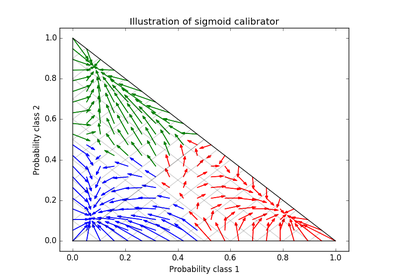Probability Calibration for 3-class classification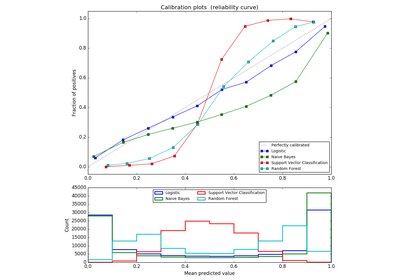Comparison of Calibration of Classifiers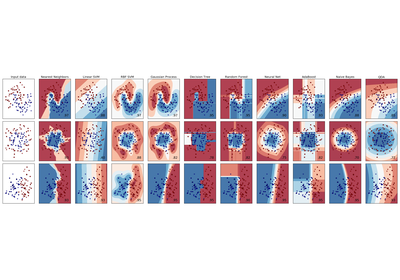Classifier comparison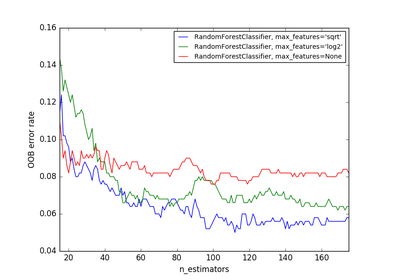OOB Errors for Random Forests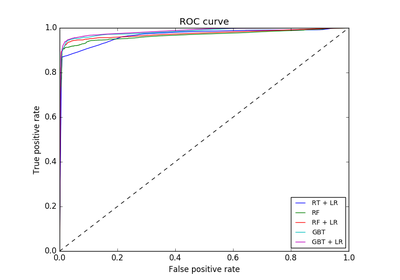Feature transformations with ensembles of trees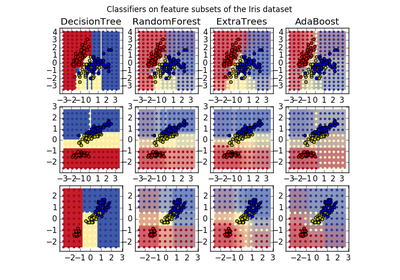Plot the decision surfaces of ensembles of trees on the iris dataset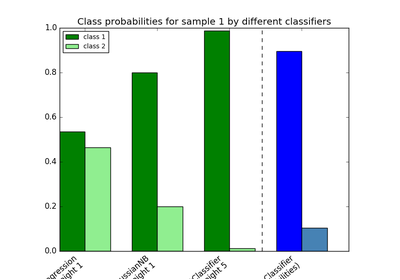Plot class probabilities calculated by the VotingClassifierComparing randomized search and grid search for hyperparameter estimationClassification of text documents using sparse features